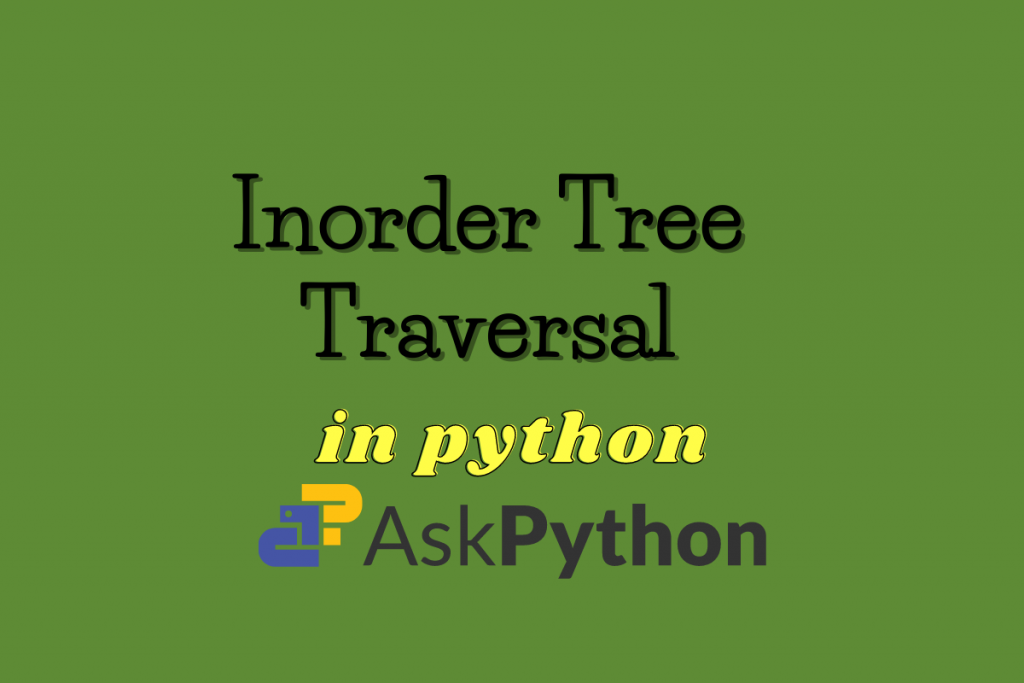# Inorder Tree Traversal in Python [Implementation]In this article, we will study the concept and algorithm for inorder tree traversal. Then we will implement the algorithm for inorder traversal in python and run it on a binary search tree.

## What is Inorder Tree Traversal?

Inorder Traversal is a depth first tree traversal algorithm. In depth first traversal, we start at the root node and then we explore a branch of the tree till end and then we backtrack and traverse another branch.

In the inorder traversal, first, we traverse the left child or left subtree of the current node and then we traverse the current node and then we traverse the right child or right subtree of the current node. We perform this operation recursively till all the nodes are traversed.We use inorder traversal to print elements of a binary search tree in increasing order.

## Inorder Tree Traversal Algorithm

Following is the algorithm for inorder traversal.

```Algorithm inorder:
Input: Reference to Root Node
Output:Prints All the nodes of the tree
Start.
1.If root is empty,return.
2.Traverse left subtree of the root.// inorder(root.leftChild)
3. Traverse the root node. //print value at node
4. Traverse the right subtree of the root.// inorder(root.rightChild)
End.

```

## Inorder Traversal Algorithm Implementation in Python

Now we will implement the above algorithm to print nodes of the following binary search tree in inorder traversal.

In the following code, first the above binary search tree has been created and then inorder traversal of the binary tree is printed.

```class BinaryTreeNode:
def __init__(self, data):
self.data = data
self.leftChild = None
self.rightChild=None

def insert(root,newValue):
#if binary search tree is empty, make a new node and declare it as root
if root is None:
root=BinaryTreeNode(newValue)
return root

#binary search tree is not empty, so we will insert it into the tree
#if newValue is less than value of data in root, add it to left subtree and proceed recursively
if newValue<root.data:
root.leftChild=insert(root.leftChild,newValue)
else:
#if newValue is greater than value of data in root, add it to right subtree and proceed recursively
root.rightChild=insert(root.rightChild,newValue)
return root

def inorder(root):
#if root is None,return
if root==None:
return
#traverse left subtree
inorder(root.leftChild)
#traverse current node
print(root.data)
#traverse right subtree
inorder(root.rightChild)

root= insert(None,15)
insert(root,10)
insert(root,25)
insert(root,6)
insert(root,14)
insert(root,20)
insert(root,60)
print("Printing values of binary search tree in Inorder Traversal.")
inorder(root)
```

Output:

```Printing values of binary search tree in Inorder Traversal.
6
10
14
15
20
25
60
```

Here, we can see that values have been printed in increasing order. So, if you are asked to print the data from a binary search tree in increasing order, you just have to perform an inorder traversal of the binary search tree.

## Conclusion

In this article, We have learned the concept of inorder tree traversal. We also studied the algorithm and implemented it in python to traverse a binary search tree and found that for a binary search tree, in order traversal prints values in increasing order. Stay tuned for more informative articles.

Happy Learning!